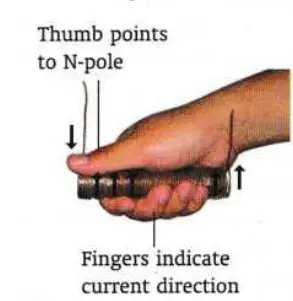# Magnetic field in solenoid – formula, direction

Last updated on December 9th, 2022 at 08:28 am

In this post, we will discuss the Magnetic field in a solenoid. We will also study the formula of the magnetic field in a solenoid and how to find out the direction of the magnetic field in a solenoid [polarity at the end of the solenoid] using the right-hand grip rule.

## Magnetic field in a solenoid | pattern of the magnetic field in a solenoid

Figure 1, shows the pattern of the magnetic field due to a current in the solenoid. The magnetic field for a solenoid has a similar pattern to the magnetic field of a bar magnet. One end of the solenoid is a north pole and the other end is a south pole.

## Formula of the magnetic field in a solenoid

Magnetic field B in a solenoid with current I can be expressed with the following formula:
B = μ0 n I
here, n = number of turns per unit length = total number of turns in the solenoid/length of the solenoid = N/L
and μ0 = permeability of air/vacuum

## Direction of the magnetic field in a solenoid

The right-hand grip rule for solenoids helps us to find out the Direction of the magnetic field in a solenoid. In other words, the polarity at the end of the solenoid can be determined using the right-hand grip rule.figure 2: The right-hand grip rule for solenoids helps us to find out the Direction of the magnetic field in a solenoid.

Right-hand grip rule for solenoids – Hold the solenoid with your right hand and your four fingers curled around the solenoid along the direction of the current. Then, the thumb will point to the end which is the north pole.